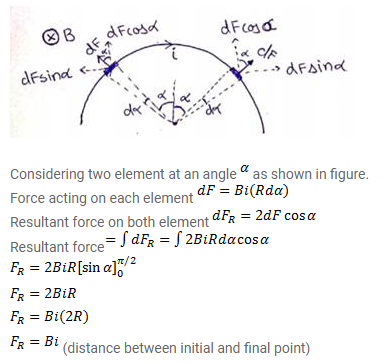# Prove that the force acting on a current-carrying wire,

Question:

Prove that the force acting on a current-carrying wire, joining two fixed points a and $b$ in a uniform magnetic field, is independent of the shape of the wire.

Solution:

Let us consider a semi-circular wire of radius $\mathrm{R}$ is placed in uniform magnetic field directed perpendicular of wire.